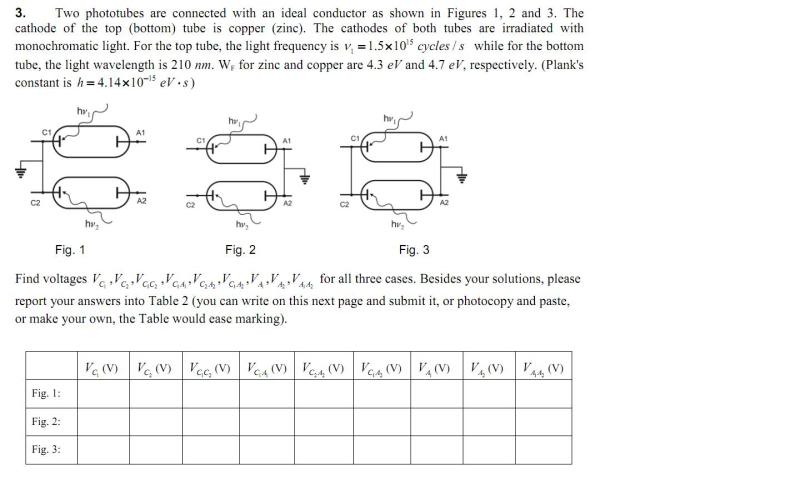# Phototubes, Voltage

dimpledur

## Homework Statement## The Attempt at a Solution

What I am wondering is if I found all of the answers for figure 1, wouldn't I just be repeating those same answers for figure 2 and 3? Or is the way it arranged important?

Here are the values I found:
Vc1=1.51V
Vc2=1.61V
Vc1c2=-0.1V
Vc1a1=1.51V
Vc2a2=1.61V
Vc1a2=1.51V
Va1=Va2=Va1a2=0

Gold Member
all arrangements are different. In fig. 1, C1 and C2 are connected, so are at same potential, but in fig.2, this is the case with A1 and A2. In fig. 3, C1 and C2 are at same potential, and A1 and A2 are also at same potential (different from C1 and C2).

dimpledur
How do you determine what potential they are at if they are at the same potential. For example, for figure one if Vc2=1.61 V and Vc1= 1.51V, would Vc1c2 just be an average of the two?

Gold Member
i think you calculated wrong. Vc1 = Vc2 (should be), and Vc1c2 = 0, as Vc1c2 = Vc1 - Vc2 (potential difference).

dimpledur
But the cathode at the bottom is struck with a different energy than the cathode at the top. Hence the difference in potentials. V=(Wf-Ephoton)V/eV

dimpledur
So if I calculated Vc1=1.51 and Vc2=1.61 would I assume they reach the same potential in figure one so Vc1=Vc2=1.56?

Gold Member
Ephoton - Wf = Eelectron, not V.

dimpledur
my textbook labels the frequency with v, which is units cycles/s

Gold Member
i am a bit unsure about Vc1 and Vc2 part, will need some time to think about it.

dimpledur
I think I got it. For figure one, Vc1 has an initial voltage due to the energy of the photon that hits it. Additionally, Vc2 has an initial voltage due to the photon that hits it as well. However, after the initial potential difference, they reach an "equilibirum" potential of 1.56. That way Vc1c2=0, Vc1=Vc2=1.56. Also, Vc1a1=Vc2a2=Vc1a2=Vc2a1=1.56 and Va1=Va2=Va1a2=0.

dimpledur
Perhaps for figure 2 Vc1=1.51 and Vc2=1.61, but since they are not connected they dont reach the same potential and Vc1c2=-0.1 Va1=Va2=Va1a2. Wouldn't the potentials of the anode in all the figures be zero?

Gold Member
but as far as i know, we never take average of potentials of two points if they are connected. so i am do not agree with above.

dimpledur
Well then I am very confused. However, wouldn't it make sense for that to occur? If there is excess charge at one point, and a conductor between two ponts, wouldn't the charge flow till eventually there was no potential difference?

Mentor
Suppose for a moment that both cathodes were made of the same material and that they were connected as in figure 1. Further suppose that only one source of light is involved. What would be the difference between a photon hitting c1 and then a photon hitting c2, and two photons hitting either c1 or c2?

dimpledur
I don't really see a difference. If electrons are leaving c1 due to a single photon hitting it, more electrons from c2 would flow via the conductor so eventually both cathodes would reach the same potential. Or am I completely off?

Mentor
I don't really see a difference. If electrons are leaving c1 due to a single photon hitting it, more electrons from c2 would flow via the conductor so eventually both cathodes would reach the same potential. Or am I completely off?

Precisely. The two cathodes are essentially one cathode when they are connected, so the work done removing the charges by the photons is "shared". The potential is the same.

dimpledur
One last question before I go. Is it true that all of the anodes have a potential of 0 regardless of the set up? for example, Vca=0, but I don't really see how Va1a2 would equal zero for figure two, because wouldn't the anodes have a different charge?

dimpledur
If only the anode is connected via a wire, wouldn't they eventually have the same charge and consequently the potential on the cathodes would change?

dimpledur
Actually, I think all the Va's will. Equal zero except for the Va1Va2 in which they're not connected. Does it make sense to say for this set up that Va1Va2=Va1c1-Va2c2